# 超基础的机器学习入门-原理篇## 基础概念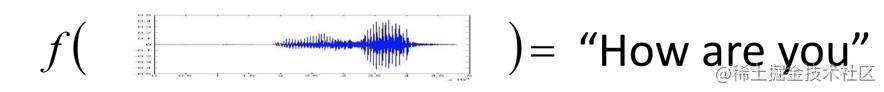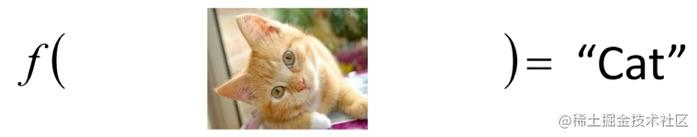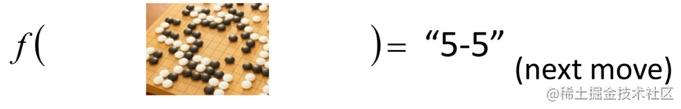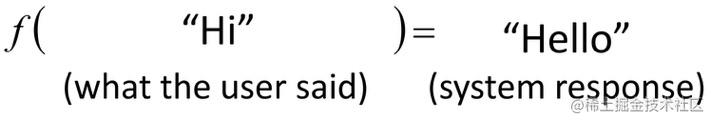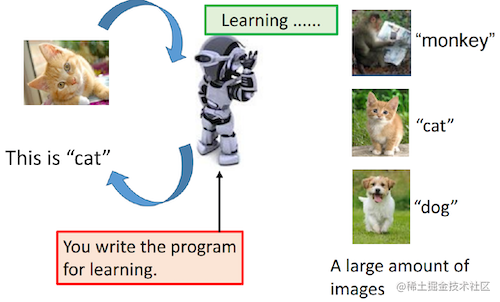## 线性模型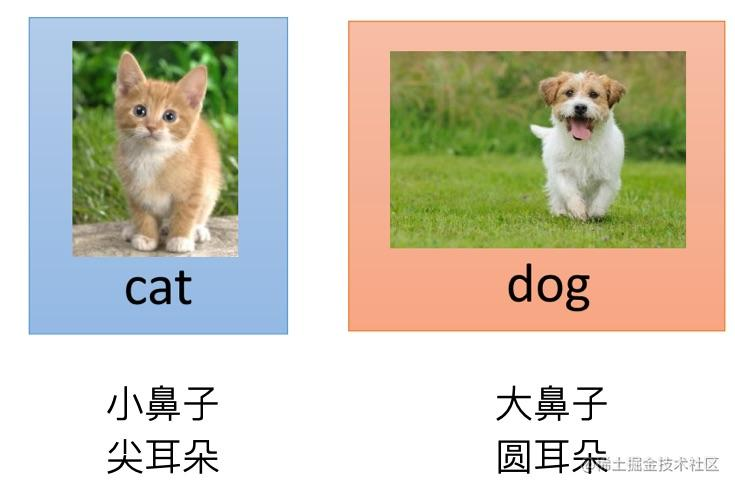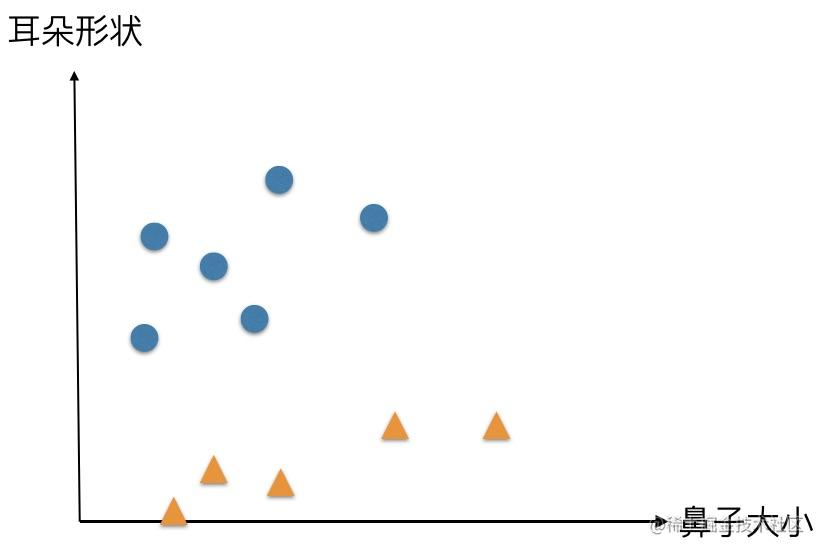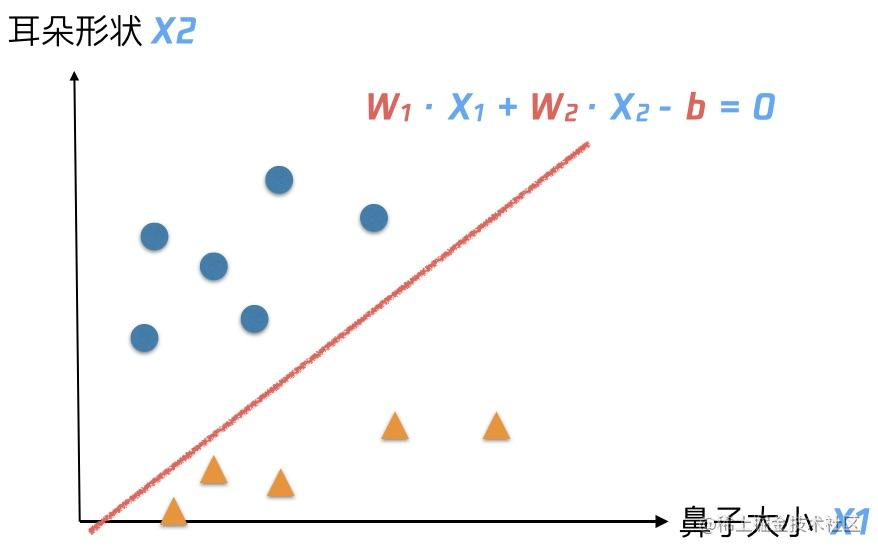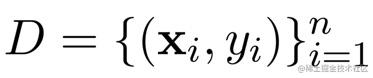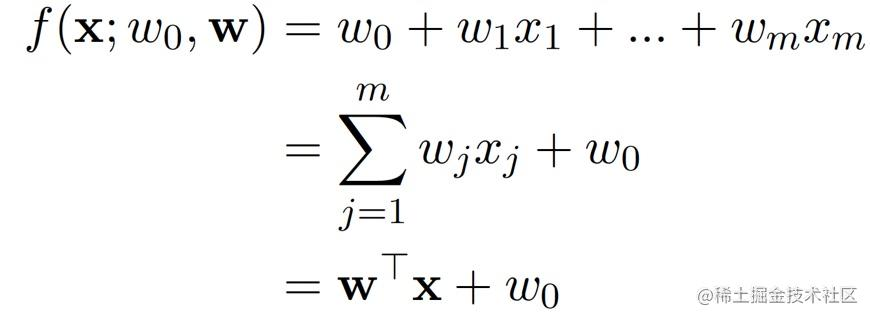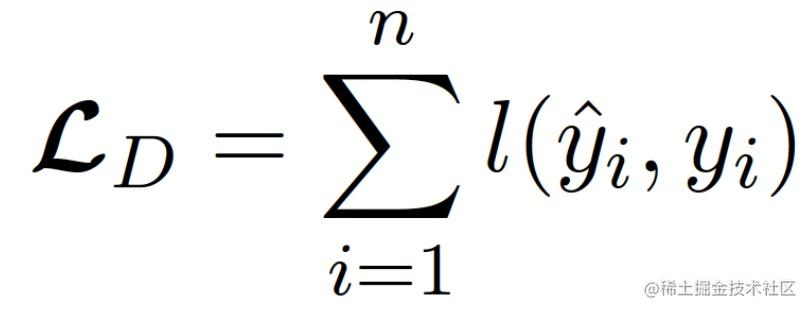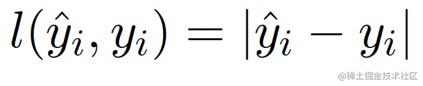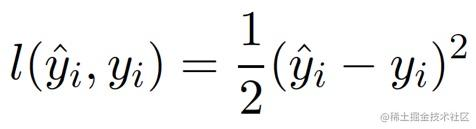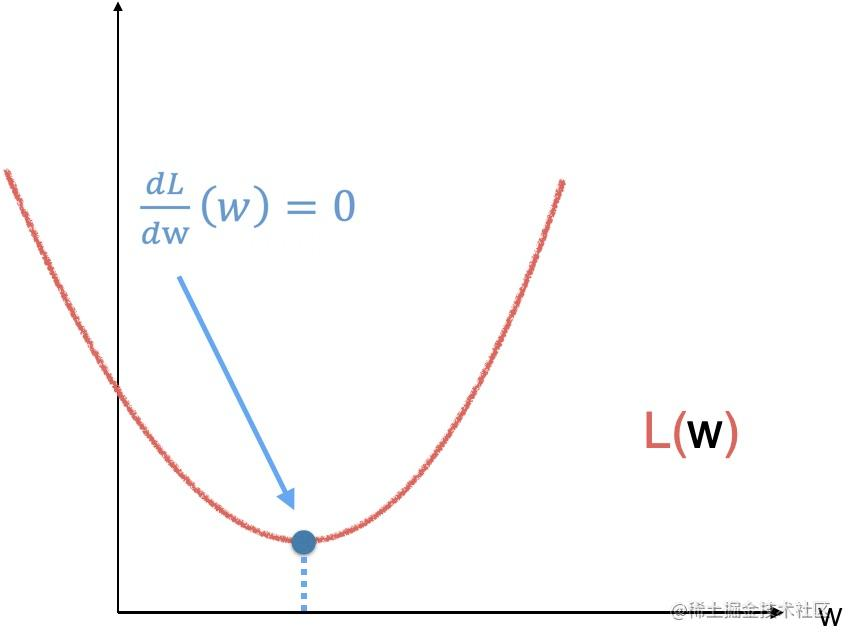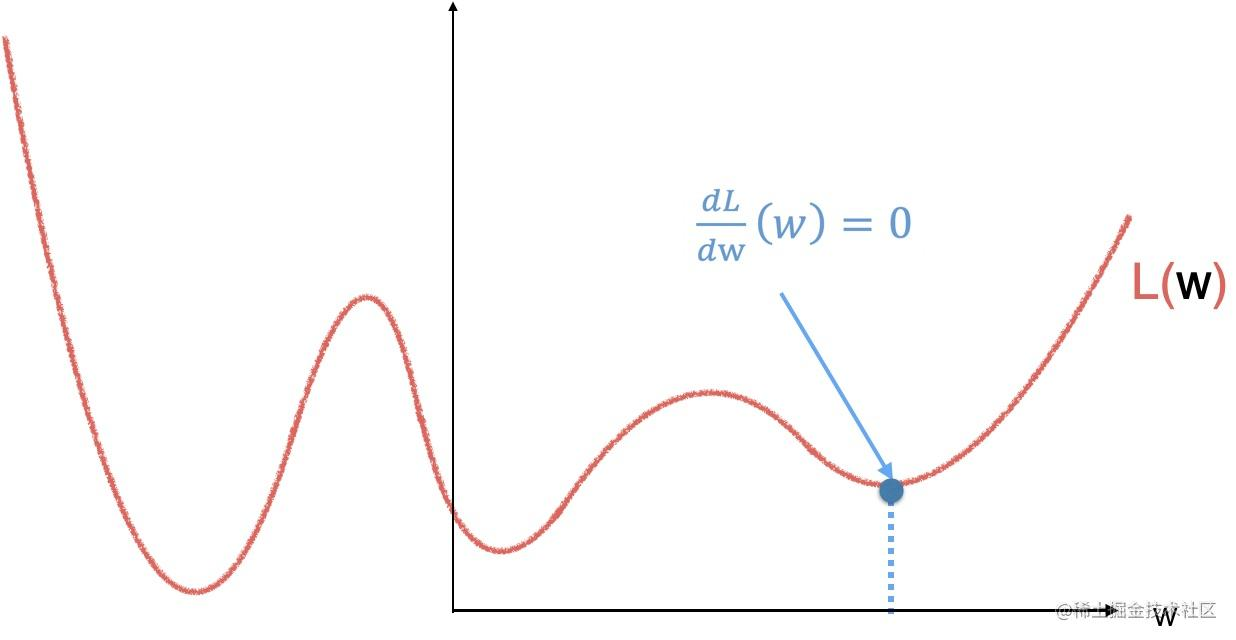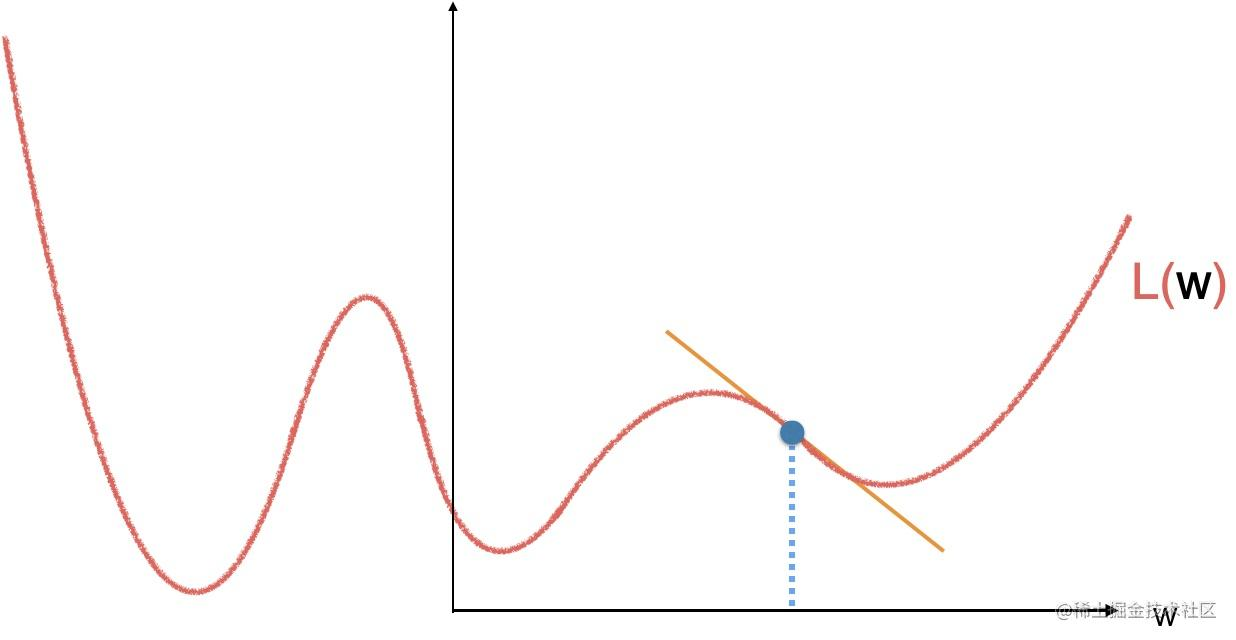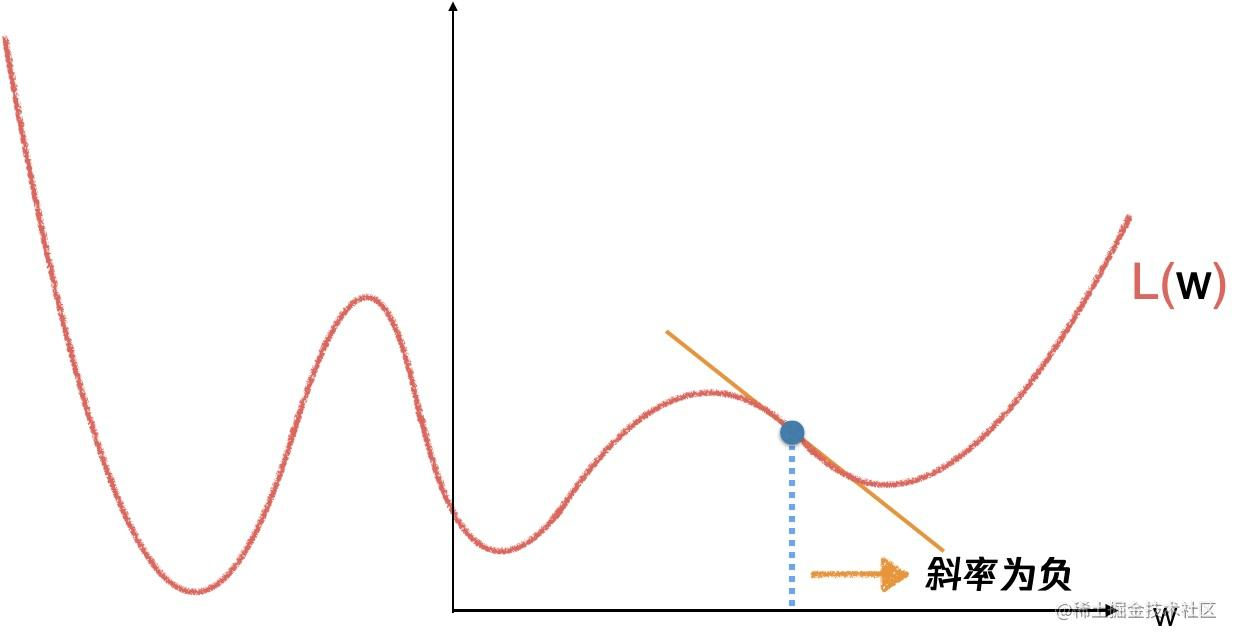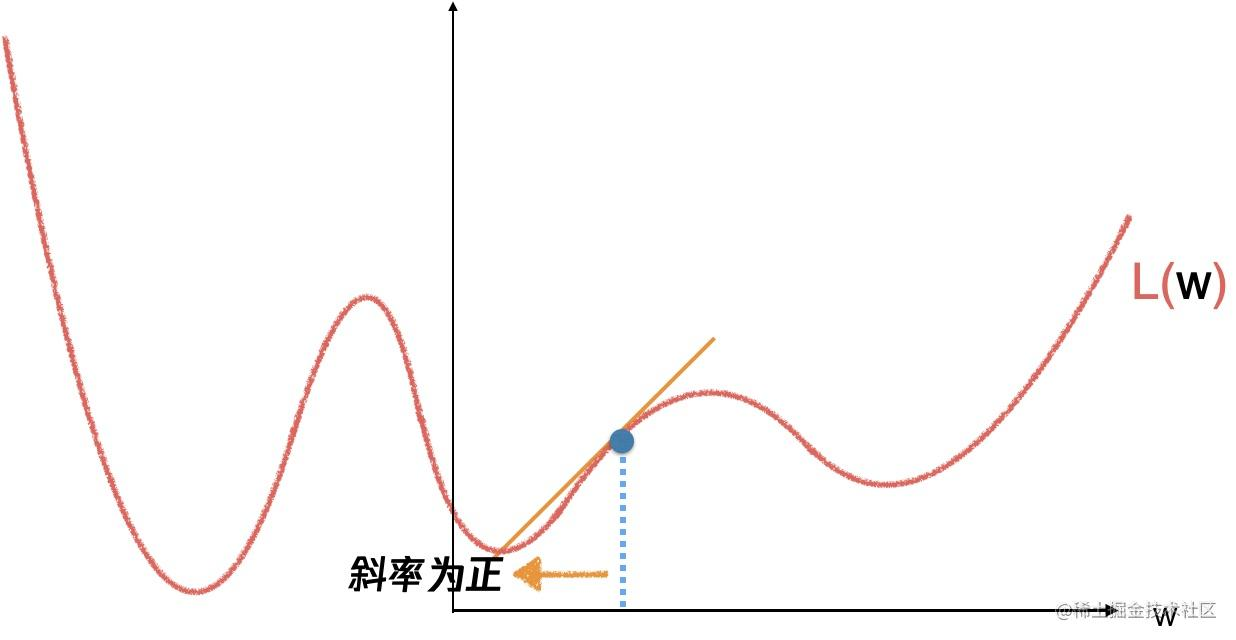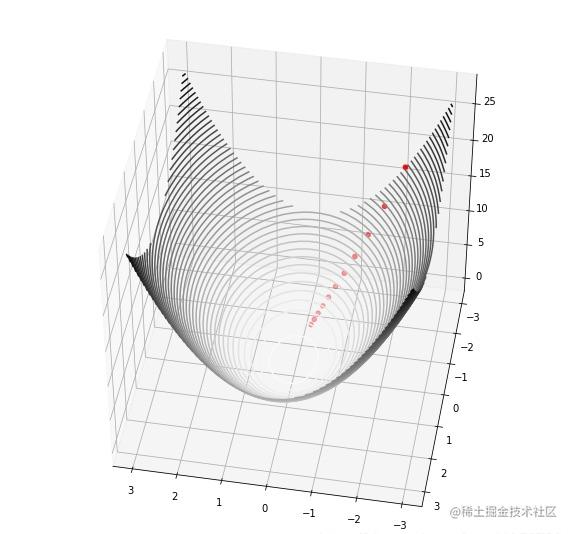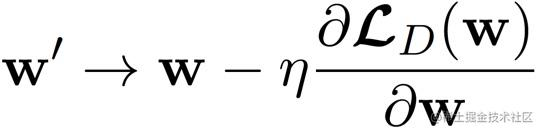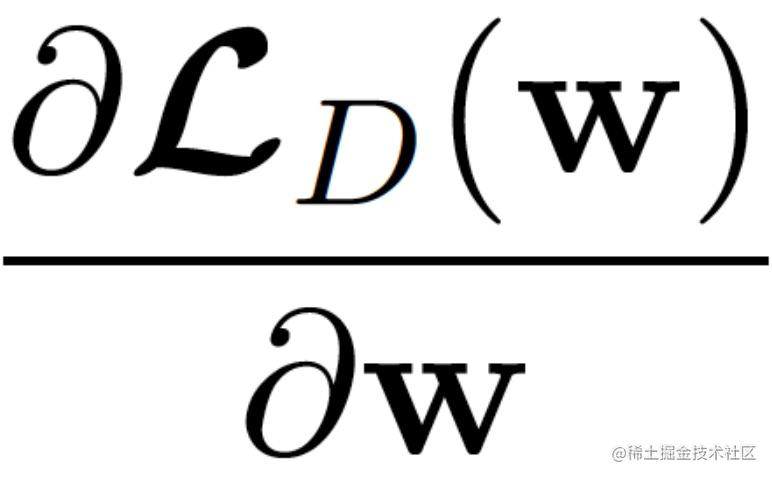`Loss function` 函数对于参数 `w` 的导数，决定了我们走的方向，那么学习率则决定了在这个方向每一小部走的距离。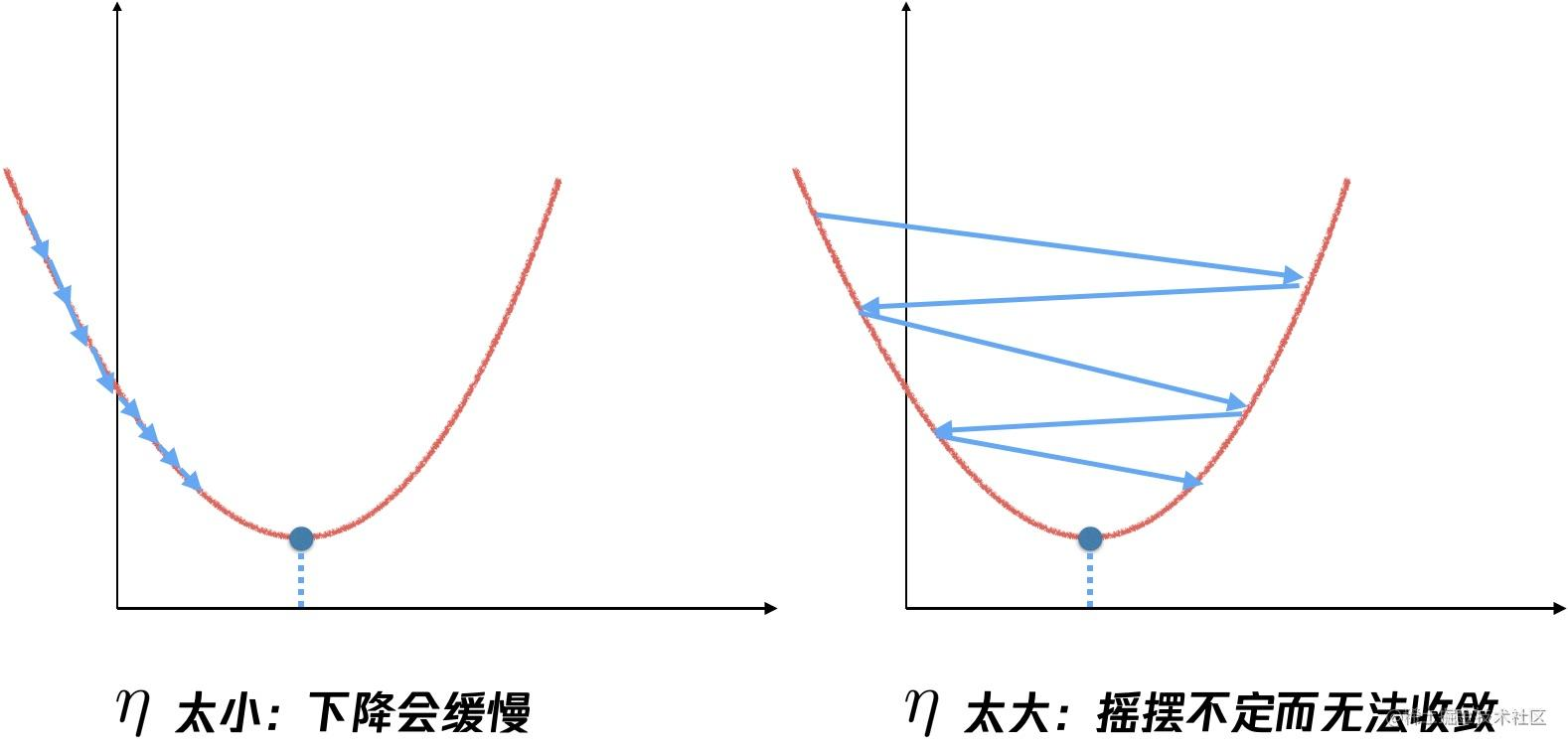`η` 太小，到达极小值的过程会非常的缓慢，而如果 `η` 太大，则会因为步伐太大，直接越过最低点。那么，`η` 的值要怎么取呢，

• 提取特征
• 设定模型
• 计算梯度，更新

``````# 损失函数
def lossFunction(x,y,w,b):
cost=np.sum(np.square(x*w+b-y))/(2*x.shape)
return cost

# 求导
def derivation(x,y,w,b):
#wd=((x*w+b-y)*x)/x.shape
wd=x.T.dot(x.dot(w)+b-y)/x.shape
bd=np.sum(x*w+b-y)/x.shape
return wd,bd

# 线性回归模型
def linearRegression(x_train,x_test,y_train,y_test,delta,num_iters):
w=np.zeros(x.shape)                                    # 初始化 w 参数
b=0                                                       # 初始化 b 参数
trainCost=np.zeros(num_iters)                             # 初始化训练集上的loss
validateCost=np.zeros(num_iters)                          # 初始化验证集上的loss
for i in range(num_iters):                                # 开始迭代啦
trainCost[i]=lossFunction(x_train,y_train,w,b)         # 计算训练集上loss
validateCost[i]=lossFunction(x_test,y_test,w,b)        # 计算测试集上loss
Gw,Gb=derivation(x_train,y_train,w,b);                 # 计算训练集上导数
Dw=-Gw                                                 # 斜率>0 往负方向走，所以需要加负号
Db=-Gb                                                 # 同上
w=w+delta*Dw                                           # 更新参数w
b=b+delta*Db                                           # 更新参数b
return trainCost,validateCost,w,b

## 多层感知机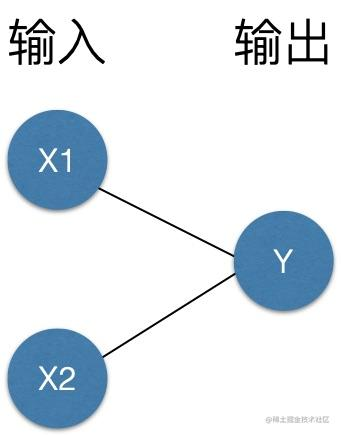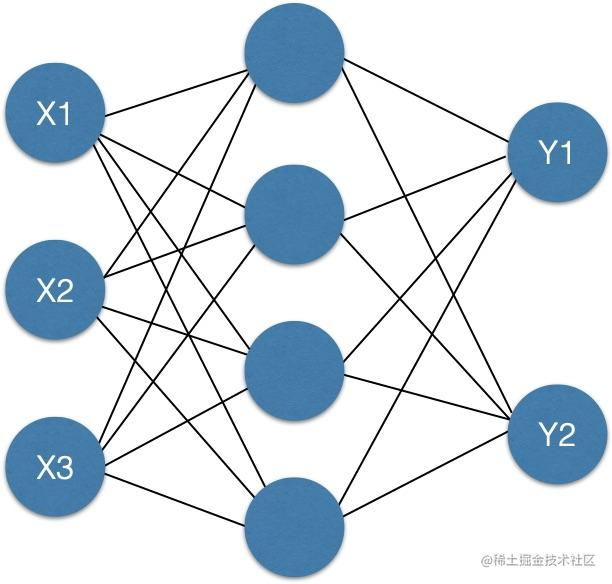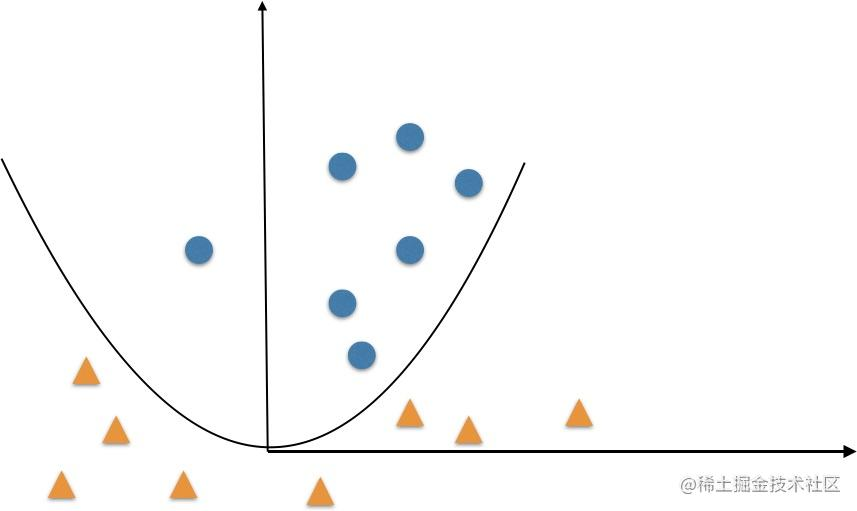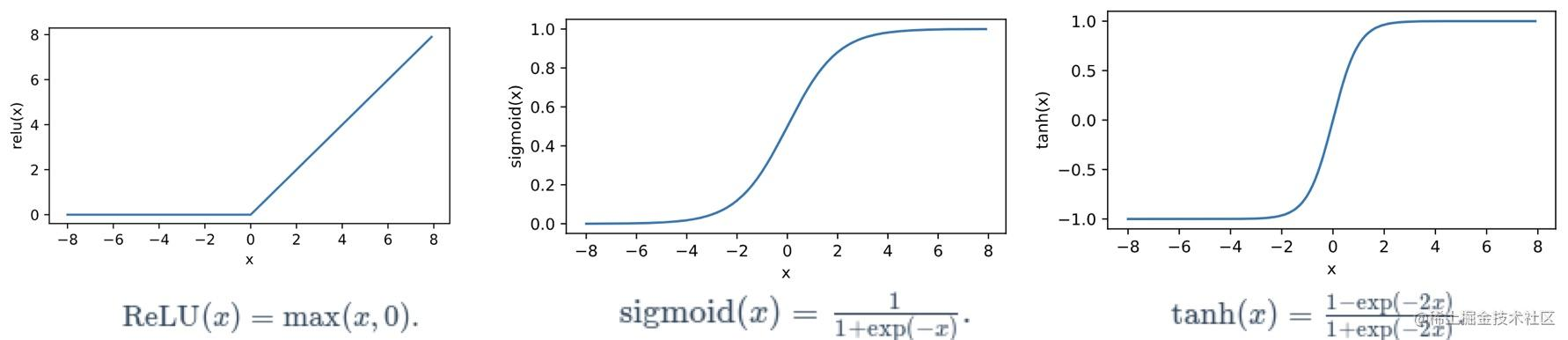`Relu` 函数只保留正数元素，清零负数元素，`sigmoid` 函数可以把元素的值变换到 `0～1` 之间，`tanh` 函数可以把元素的值变换到 `-1～1` 之间。其中用到最广泛的是看上去最简单的 `Relu``Relu`函数就好比人脑神经元，达到神经元的刺激阈值就输出，达不到阈值就置零。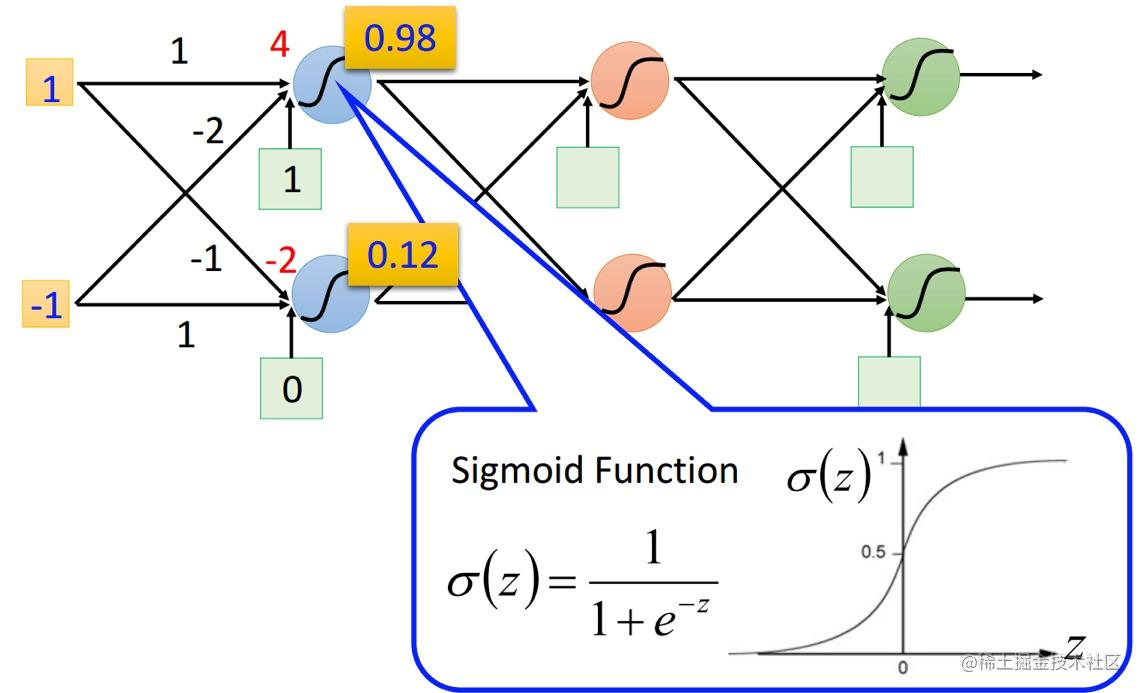## 中途小结

``````for i in 迭代次数：
loss = 预测值和真实值的差距
d = loss 对 w求导
w = w - d * 学习率

## 卷积神经网络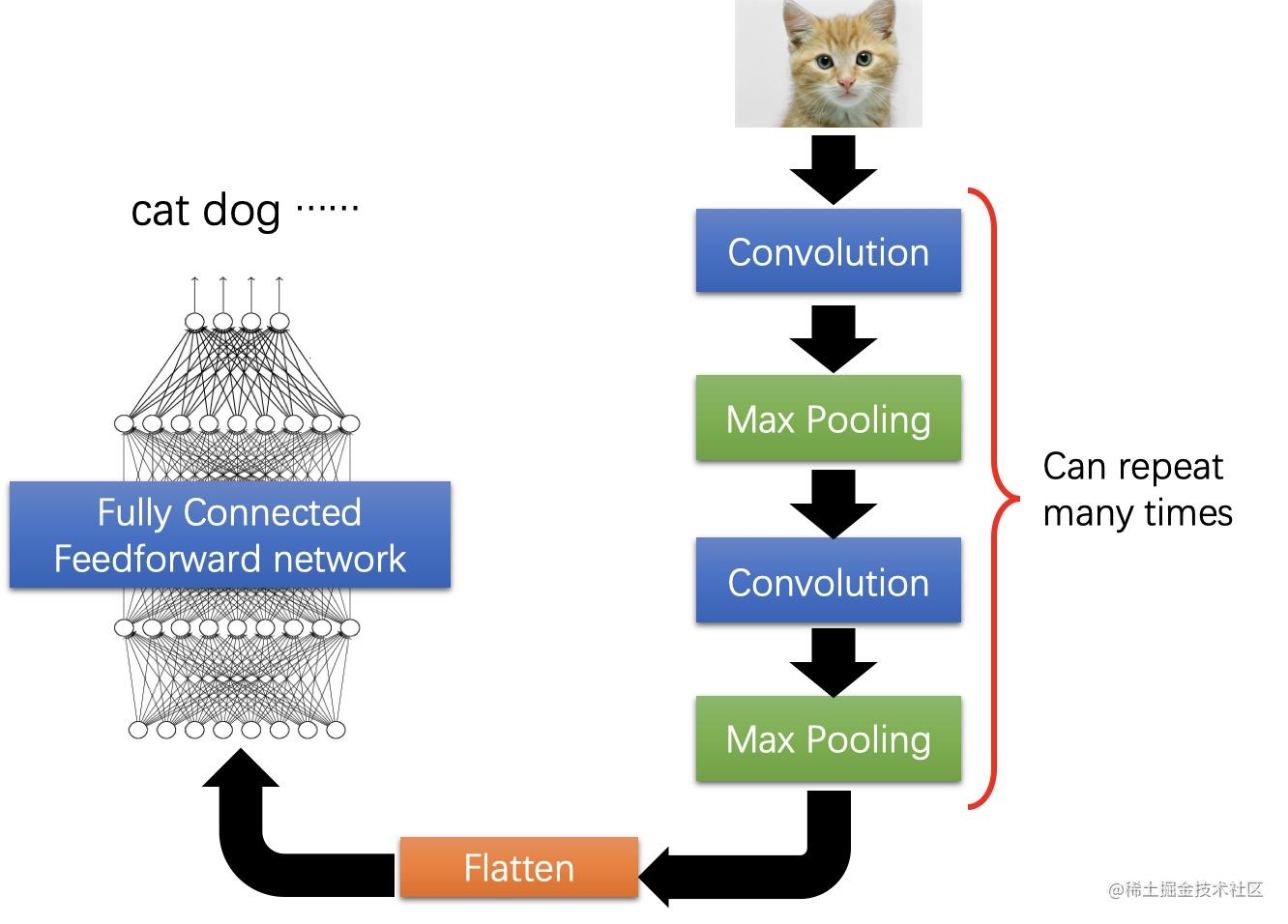• 卷积层
• 池化层
• 全连接层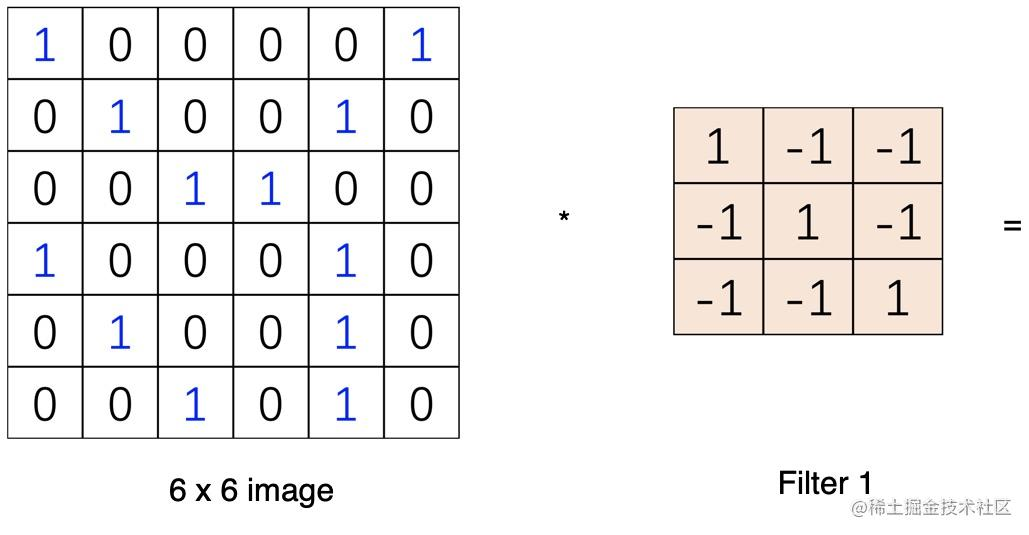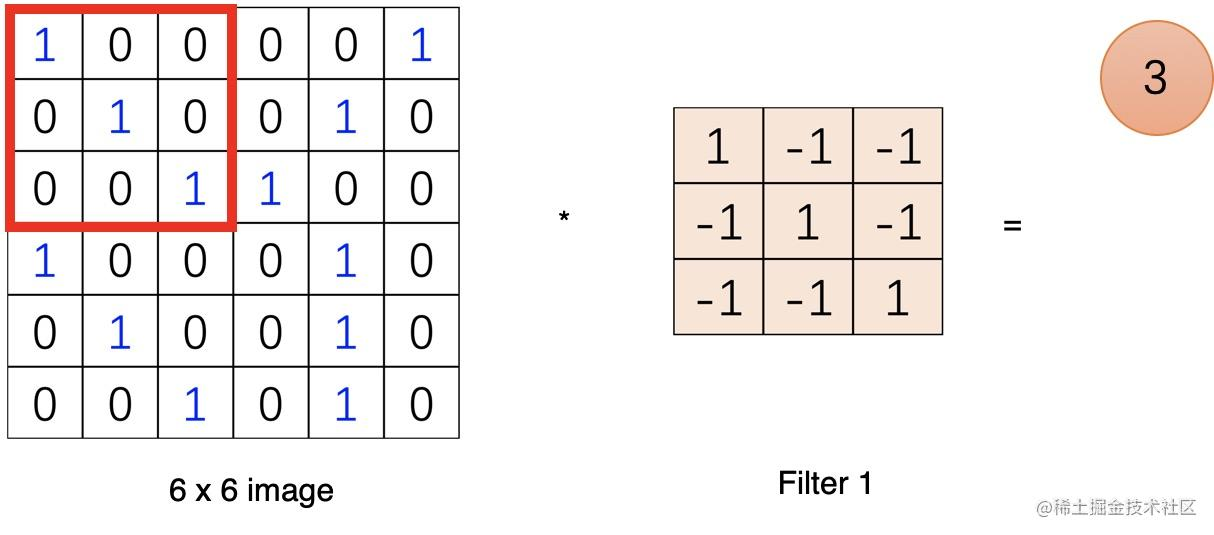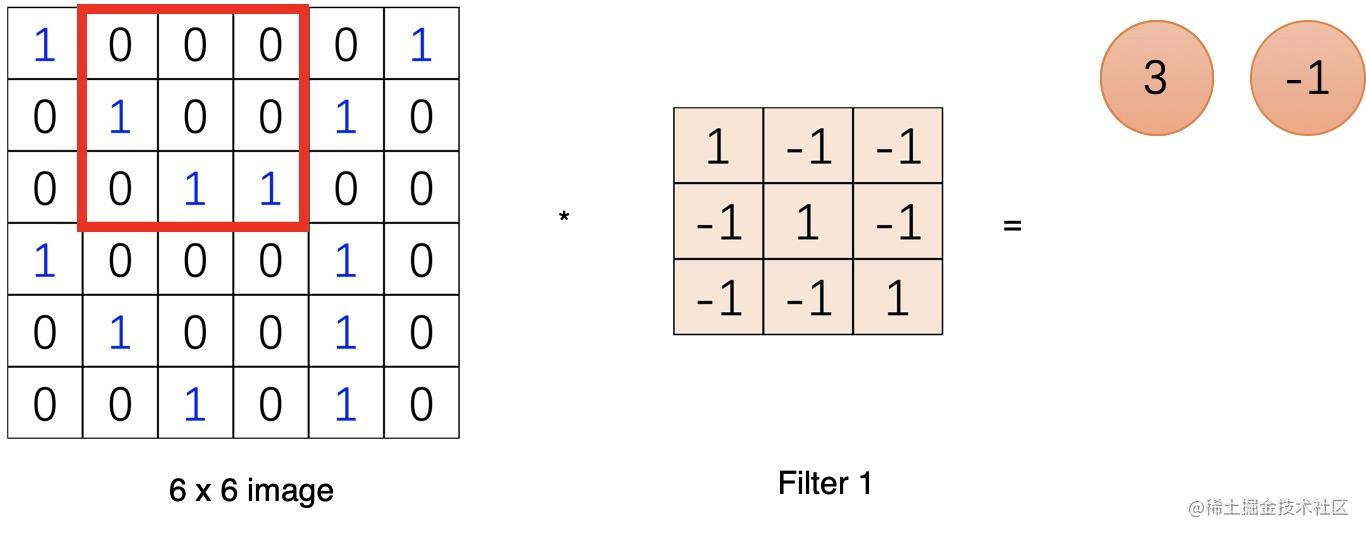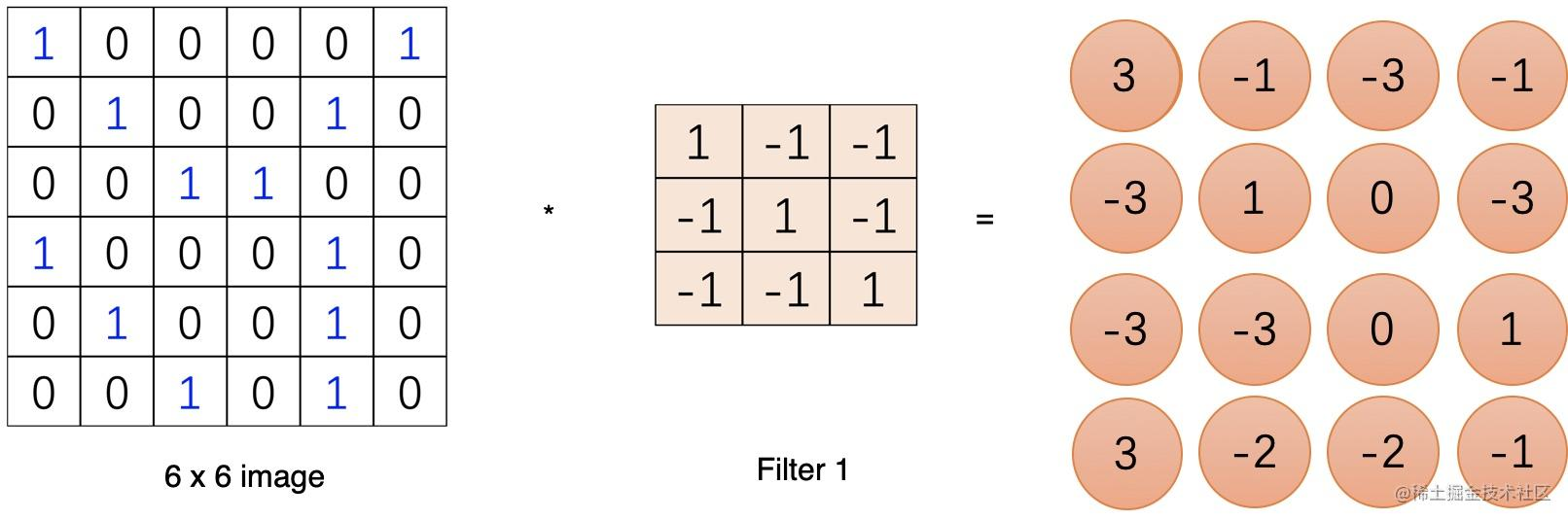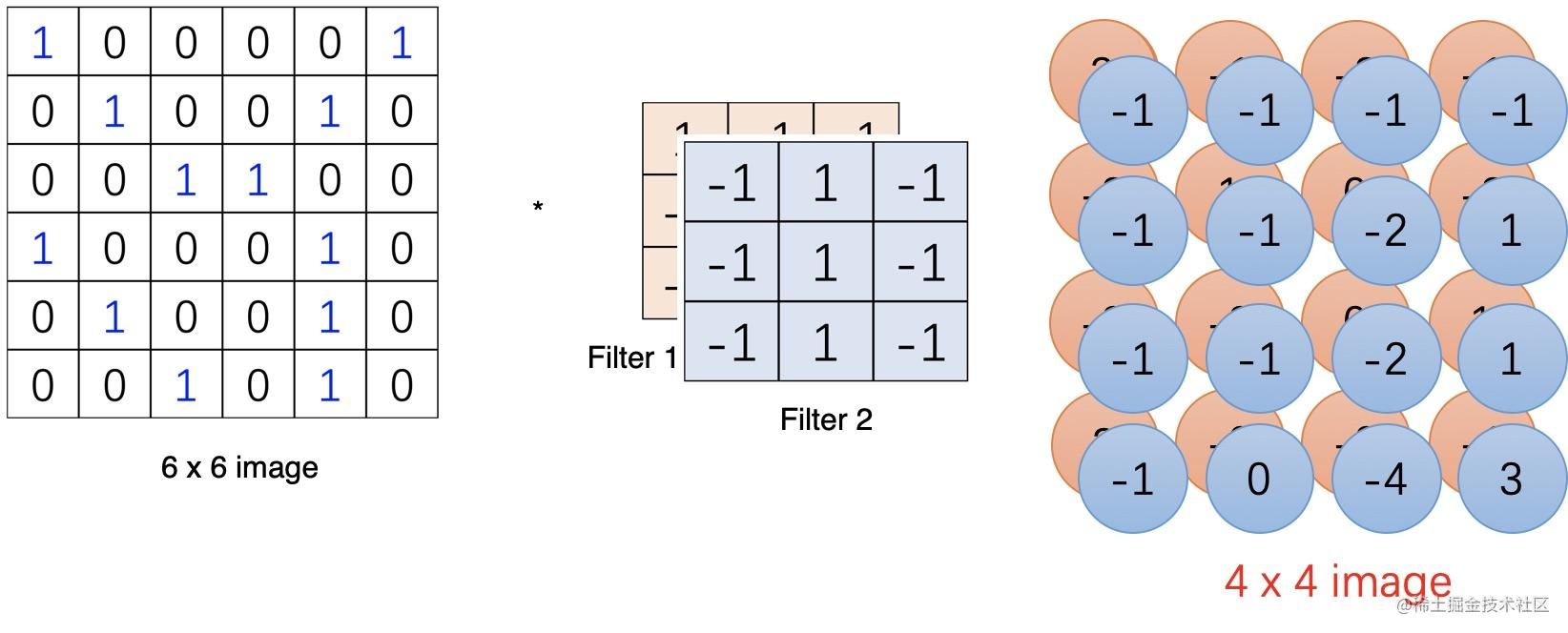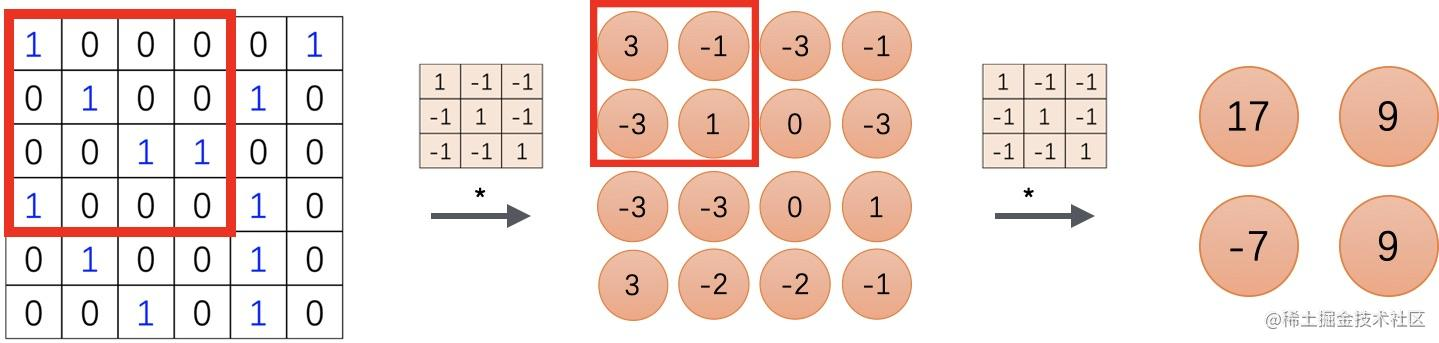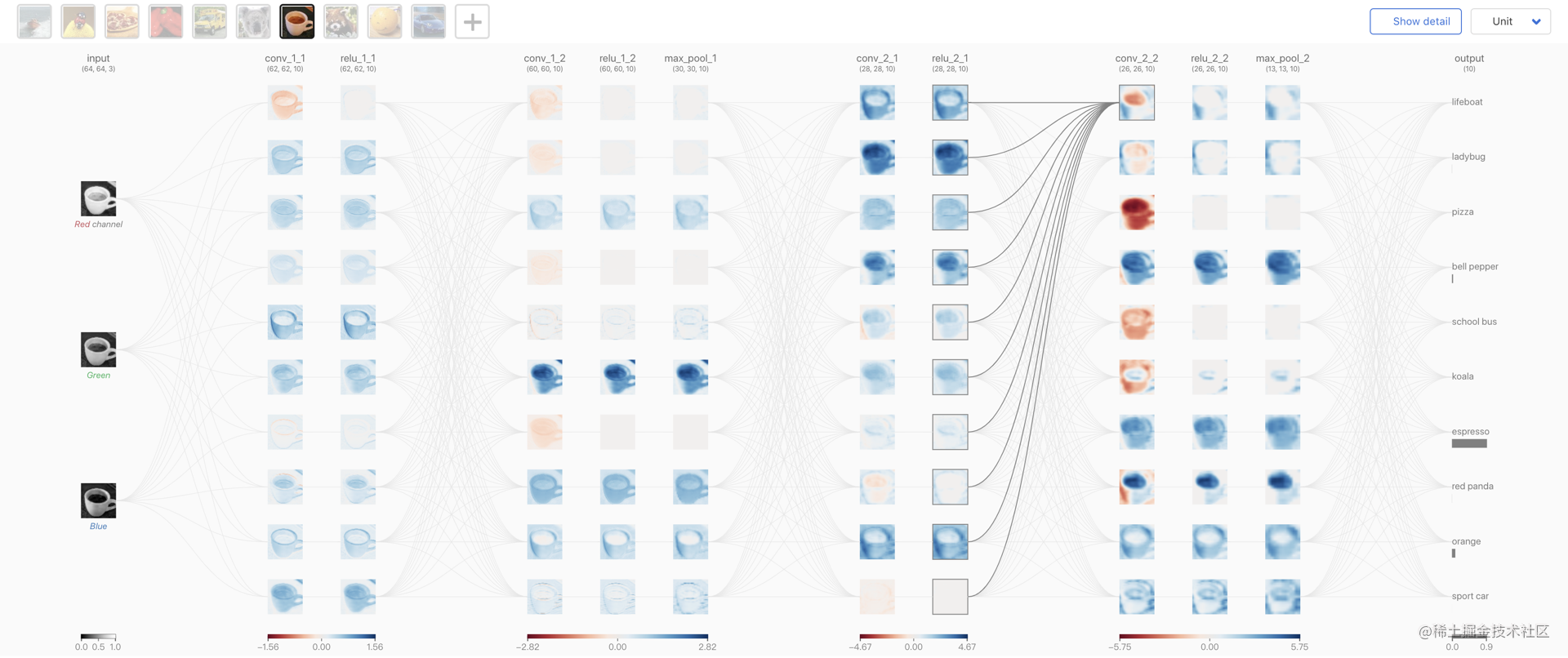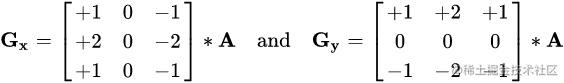`sobel``x` 方向的边缘检测计算结果如下所示：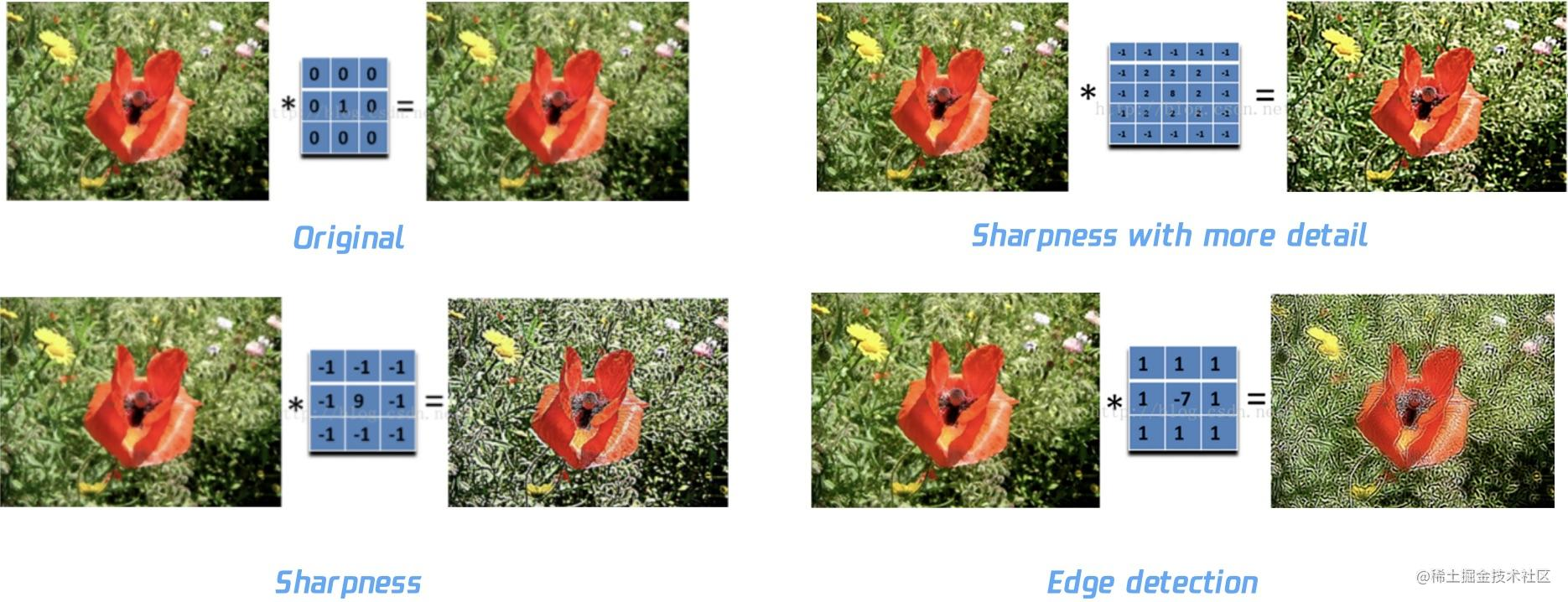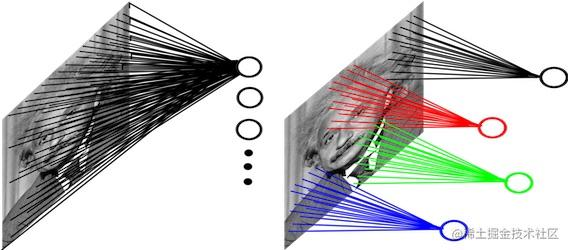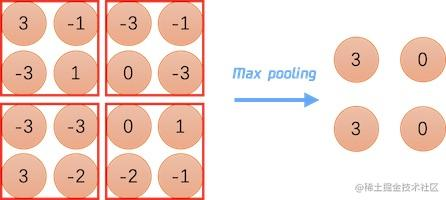## 最后的最后

Deco 智能代码项目是凹凸实验室在「前端智能化」方向上的探索，我们尝试从设计稿生成代码（DesignToCode）这个切入点入手，对现有的设计到研发这一环节进行能力补全，进而提升产研效率。其中使用到不少AI能力来实现设计稿的解析与识别。